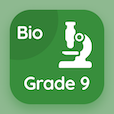Cambridge Online Courses (GCSE)

O Level Physics Quizzes

O Level Physics Quiz PDF - Complete

# Temperature Scales Quiz Questions Online p. 178

Learn Temperature Scales quiz questions and answers, temperature scales MCQ with answers PDF 178 to study O Level Physics course online. Temperature in Physics trivia questions, temperature scales Multiple Choice Questions (MCQ) for online college degrees. "Temperature Scales Quiz" PDF Book: boiling and condensation, latent heat, thermal properties, melting and solidification, temperature scales test prep for online colleges for science.

"If an unmarked mercury-in-glass thermometer has l100 23 cm and l0 is 8 cm, if the lθ is 5 cm the temperature of the thermometer would be" MCQ PDF: −20 °c, −10 °c, −30 °c, and −40 °c for college entrance exams. Study temperature in physics questions and answers to improve problem solving skills for online associates degree.

## Quiz on Temperature Scales MCQs

MCQ: If an unmarked mercury-in-glass thermometer has l100 23 cm and l0 is 8 cm, if the lθ is 5 cm the temperature of the thermometer would be

−10 °C
−20 °C
−30 °C
−40 °C

MCQ: Melting point of wax is

37 °C
47 °C
57 °C
67 °C

MCQ: Formula of specific heat capacity is

c = C/Δθ
c = Q/Δθ
c = C/m
c = C × m

MCQ: Unit of specific latent heat of vaporization is

Kelvin per kilogram
Pascal per kilogram
Joules per Kilogram
Ohms per kilogram

MCQ: When a pure substance changes its state from liquid to gas upon heating, the process is termed as

boiling
solidification
condensation
sublimation

### More Quizzes from O Level Physics Course